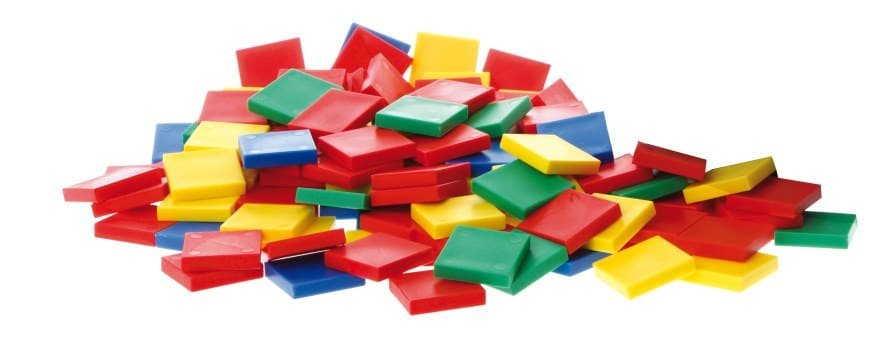# Colour Tiles

#### • probability:

Colour tiles are 25 mm (1 in) square tiles (5 mm thick) that come in four colours. They are the ideal manipulative for developing measurement concepts such as area. Area is simply a measure of covering. Square tiles are the ideal unit for covering an area as they tessellate, that is, tile without a gap.

### Videos

0:00 – Intro
2:00 – Description of Colour Tiles
4:20 – Odd & Even Numbers
5:45 – Square Numbers
7:00 – Symmetry
8:30 – Area & Perimeter
14:30 – Graphing
19:30Pattern & Algebra
20:00Positional Language, Number, Mathematical Vocabulary
24:00 – Problem Solving

### Mathematical Language

Area, chance, maybe, pattern, perimeter, possibly, probably.
Note that many students mix up the idea of area and perimeter. The word perimeter is made up of two base words, meter (to measure) and peri (around). Sharing the origin of the word perimeter may help.

### Using Colour Tiles

Simple Counting

#### Pattern

Colour tiles may be used to illustrate the relationship between patterns. For example, the square numbers may be depicted using colour tiles.

#### Fractions

Fraction understanding may be fostered by asking students to build a shape that is one-fourth red and three-fourths blue.

#### Probablitity

Colour tiles may be placed into a drawstring bag and tiles drawn out to conduct probability experiments and collect data.

#### Measurement

Using tiles to cover an area

#### Geometry

Symmetry